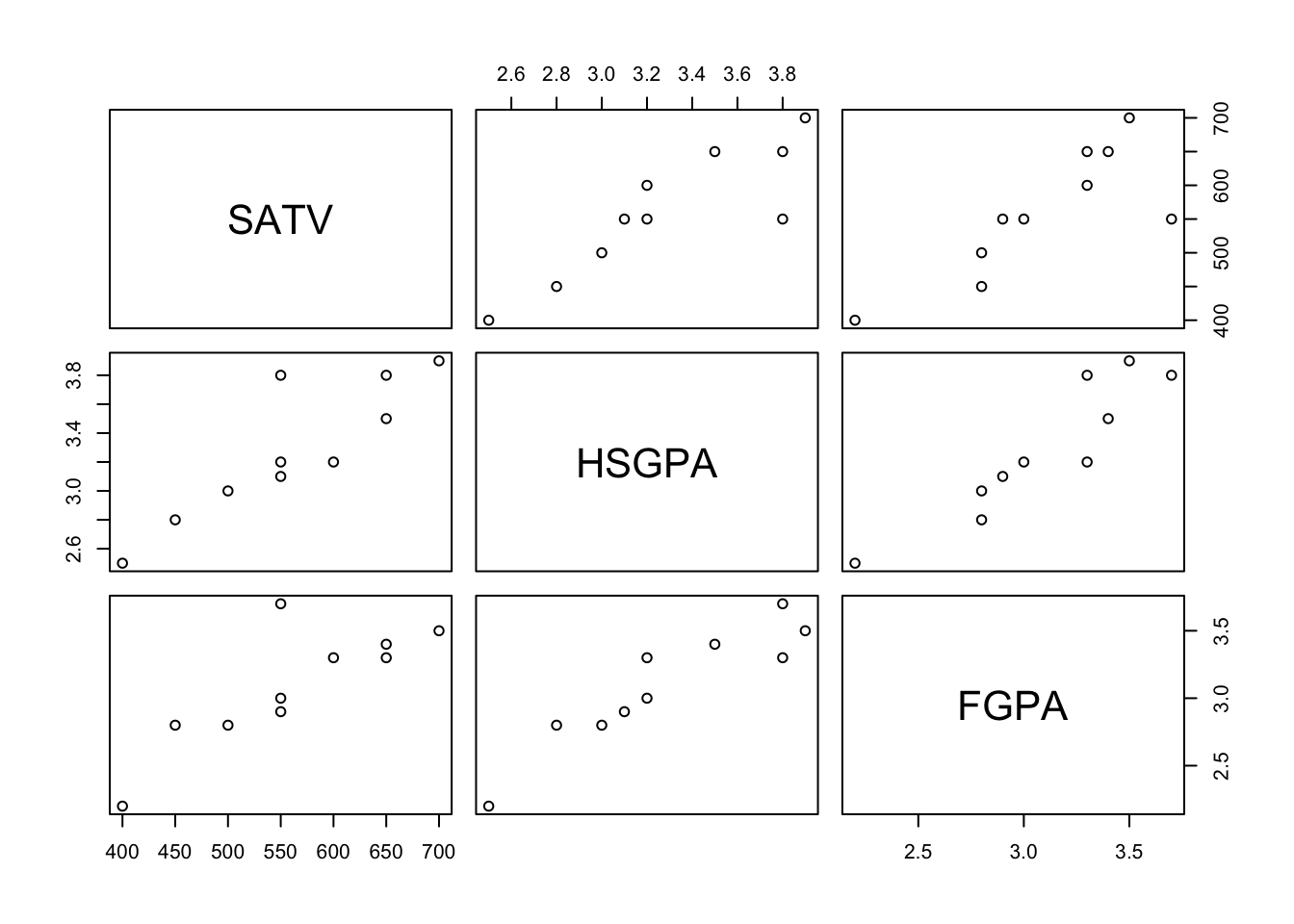## Test scores and grades example

library(psych) # for descriptive statistics
library(ppcor) # this pacakge computes partial and semipartial correlations.
## Loading required package: MASS

The example comes from my PowerPoint slideshow. Suppose we have SAT-Verbal scores and both high school and college freshman grade point averages. We might want to estimate the relations among some of these while holding others constant through statistical control.

The data follow.

options(digits = 4)
SATV <-  c(500, 550, 450, 400, 600, 650, 700, 550, 650, 550)
HSGPA <- c(3.0, 3.2, 2.8, 2.5, 3.2, 3.8, 3.9, 3.8, 3.5, 3.1)
FGPA <-  c(2.8, 3.0, 2.8, 2.2, 3.3, 3.3, 3.5, 3.7, 3.4, 2.9)
scholar <- data.frame(SATV, HSGPA, FGPA) # collect into a data frame
describe(scholar) # provides descrptive information about each variable
##       vars  n   mean    sd median trimmed    mad   min   max range  skew
## SATV     1 10 560.00 93.69 550.00  562.50 111.19 400.0 700.0 300.0 -0.17
## HSGPA    2 10   3.28  0.46   3.20    3.30   0.52   2.5   3.9   1.4 -0.08
## FGPA     3 10   3.09  0.44   3.15    3.12   0.44   2.2   3.7   1.5 -0.50
##       kurtosis    se
## SATV     -1.27 29.63
## HSGPA    -1.43  0.15
## FGPA     -0.77  0.14

We have have 10 observations with no missing data in our illustration. A real study would have a much larger sample size.

Basic information about the relations among the variables.

corrs <- cor(scholar) # find the correlations and set them into an object called 'corrs'
corrs                 # print corrs
##         SATV  HSGPA   FGPA
## SATV  1.0000 0.8745 0.8144
## HSGPA 0.8745 1.0000 0.9226
## FGPA  0.8144 0.9226 1.0000
pairs(scholar)        # pairwise scatterplotsAll of the correlations are quite large.

Suppose we have three variables, X, Y, and Z. Partial correlation holds constant one variable when computing the relations two others. Suppose we want to know the correlation between X and Y holding Z constant for both X and Y. That would be the partial correlation between X and Y controlling for Z. Semipartial correlation holds Z constant for either X or Y, but not both, so if we wanted to control X for Z, we could compute the semipartial correlation between X and Y holding Z constant for X.

### Example Partial Correlation

Suppose we want to know the correlation between the two grade point averages controlling for SAT-V. How highly correlated are these after controlling for scholastic ability? This would get at the importance of motivation, study habits, and other noncognitive variables. The R syntax using ‘pcor’ is

pcor.test(HSGPA,FGPA,SATV)
##   estimate p.value statistic  n gp  Method
## 1   0.7476 0.02057     2.978 10  1 pearson

The estimate is about .75, which is still a large correlation. Note that the syntax requires the two focal variables (X, Y) be listed first, and that the control variable (Z) be listed last. There can be more than one control variable.

There are a couple of other ways to estimate this correlation. Using residuals from regression is an instructive way to consider it, although you wouldn’t usually compute it this way in practice. A second way is to use observed correlations that you might have found in a table in a manuscript so that you do not have access to the raw data.

The regression approach:

reg1 <- lm(HSGPA ~SATV)   # run linear regression
resid1 <- resid(reg1)     # find the residuals - HSGPA free of SATV
reg2 <- lm(FGPA ~ SATV)   # second regression
resid2 <- resid(reg2)     # second set of residuals - FGPA free of SATV
cor(resid1, resid2)       # correlation of residuals - partial correlation
##  0.7476

As you can see, the resulting correlation is the same as was computed previously using pcor. Such equivalence shows the connection between regression and partial correlation.

The second method is to compute the partial from observed correlations (see my slideshow for the formulas).

r12.3 <- (corrs[2,3]-corrs[2,1]*corrs[3,1])/(sqrt(1-corrs[2,1]^2)*sqrt(1-corrs[3,1]^2))
r12.3 
##  0.7476

As you can see, the result is the same as the two previous calculations. Note that ‘corrs’ is the correlation matrix computed at the beginning of this file. Because we are dealing with correlations, we don’t have to know the means and standard deviations of the variables.

### Example Semipartial Correlation

Suppose that we want to control high school GPA for SAT-V, but we want no statistical control applied to freshman GPA. This would address how well high school GPA (HSGPA) correlated with college freshman GPA (FGPA) above and beyond SAT-V.

The R syntax using ‘spcor’ is:

spcor.test(FGPA,HSGPA,SATV)
##   estimate p.value statistic  n gp  Method
## 1   0.4338  0.2434     1.274 10  1 pearson

Note that the statistical control is applied to the right-hand partner of the first two variables (here HSGPA), and the control variable is the last in the list (here SATV).

Note also that the semipartial correlation is smaller than was the partial correlation. That part of freshman GPA that is attributable to cognitive ability was removed in the partial correlation, but it remains in the semipartial, so the resulting correlation is smaller in the semipartial.

We can also compute the semipartial using regression residuals and using formulas for the observed correlations, just as we could for the partial correlation.

Regression estimates:

cor(FGPA, resid1)
##  0.4338

As you can see, the result is the same as using ‘spcor’ (resid1 was computed earlier; see the code above).

We can also compute the semipartial from observed correlations:

r1_2.3_ <- (corrs[2,3]-corrs[2,1]*corrs[3,1])/(sqrt(1-corrs[2,1]^2))
r1_2.3_
##  0.4338

As you can see, the result is the same as the earlier computations. The matrix ‘corrs’ was computed at the beginning of the file.